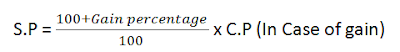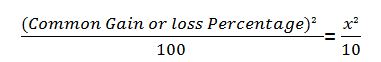New Students Offer - Use Code HELLO

# Profit and loss Formulas

Profit and loss problems involve various terms like Cost price, Selling price, Marked price and Discount etc. Basically, it is a difference between selling price and cost price.

Cost price is the price paid to purchase an article or a product or we can say it is a cost incurred in manufacturing an article.

Selling price is the price at which a product is sold.

## 6 Commonly used Profit and loss Formulas :

### 1) Calculation of Profit:

Profit or gain = Selling price(S.P) - Cost price(C.P)
in other ,
⇒ Selling Price = Cost Price + Profit

### 2) Calculation of Loss

Loss = Cost price - Selling price

### 3) Calculation of Gain percentage(%)### 4) Calculation of Loss percentage(%)### 5) Finding relationship between selling price and cost price:For Example: If an article is sold at a gain of 27%, then by using the first formula, you can find that S.P. is 127% of C.P.
Similarly, If an article is sold at loss of 18%, then by using the second formula, you can find that S.P. is 82% of C.P.

### 6) Finding Combined Profit/Loss on sale of Two products

If a person sells two commodities at same prices. On one he gains x% and loses x% on another, then as a whole he will be in loss and the loss percentage will be equal to:Note: Here is an example to find gain in case of dishonesty.
Problem 1: A dishonest dealer professes to sell his goods at cost price but he uses a weigh 960 grammes for 1 kg. How to calculate the gain percentage?
Solution: